# 1. Air in a cylinder with a volume of 500 cm3 is compressed by a piston...

1. Air in a cylinder with a volume of 500 cm3 is compressed by a piston to a volume of 150 cm3. If the initial air pressure is 5 x 104 Pa (N/m2), what is the pressure in the cylinder after compression? (Assume that this is a slow process, and that T remains constant.)

8.  The sample of gas in Question 2 is contained in a rigid closed container. If the pressure of the gas is 1013 mb at 20°C, what is the pressure of the gas after T was doubled from 0°C?

( Question 2: A temperature of a sample of gas at 0°C is doubled. What is the final temperature of the gas in °C? )

This Homework Help Question: "1. Air in a cylinder with a volume of 500 cm3 is compressed by a piston..." No answers yet.

We need 10 more requests to produce the answer to this homework help question. Share with your friends to get the answer faster!

0 /10 have requested the answer to this homework help question.

Once 10 people have made a request, the answer to this question will be available in 1-2 days.
All students who have requested the answer will be notified once they are available.

#### Earn Coin

Coins can be redeemed for fabulous gifts.

Similar Homework Help Questions
• ### Q-1 Air at 1 atm and 20 0C occupies an initial volume of 1000 cm3 in...

Q-1 Air at 1 atm and 20 0C occupies an initial volume of 1000 cm3 in a cylinder. The air is confined by a piston which has a constant restraining force so that the gas pressure always remains constant. Heat is added to the air until its temperature reaches 260 0C. Calculate (a) the heat added (b) the work done by the gas, (c) the change in internal energy of the gas. Q-2 An ideal gas is contained in a...

• ### .    BB guns are typically air rifles employing compressed air in a small (1 cm3) cylinder...

.    BB guns are typically air rifles employing compressed air in a small (1 cm3) cylinder to propel a projectile.   The typical pressure inside the cylinder when the gun is ready to fire is around 1 MPa. The BBs have a mass of 5 g, and they act as the piston head as they are held by a pin (attached to the trigger). When the trigger is pulled, the pin is released and the air expands in an isothermal process...

• ### Air is being compressed in a piston-cylinder device in a reversible and adiabatic manner. During the...

Air is being compressed in a piston-cylinder device in a reversible and adiabatic manner. During the process both the temperature and pressure of the gas increase, while the volume is decreasing. If the initial volume and temperature of the air are 3 m3 and 13 °C, respectively, and the final volume is measured to be 0.3 m3, the final temperature of the air is

• ### Air in a piston-cylinder device is compressed from 27°C and 100 kPa to 900 kPa by...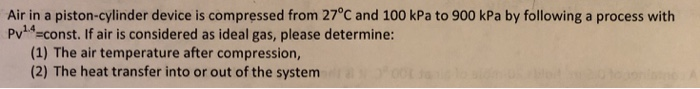Air in a piston-cylinder device is compressed from 27°C and 100 kPa to 900 kPa by following a process with Pv14-const. If air is considered as ideal gas, please determine: (1) The air temperature after compression, (2) The heat transfer into or out of the system

• ### In an experiment to simulate conditions within an automobile engine, 0.175 mol of air at a temper...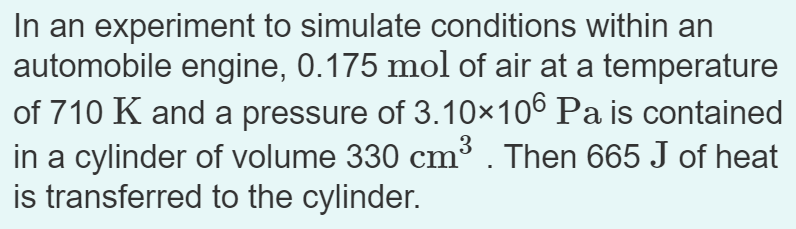In an experiment to simulate conditions within an automobile engine, 0.175 mol of air at a temperature of 710 K and a pressure of 3.10x106 Pa is contained in a cylinder of volume 330 cm3. Then 665 J of heat is transferred to the cylinder. If the volume of the cylinder is constant while the heat is added, what is the final temperature of the air? Assume that the air is essentially nitrogen gas If instead the volume of the...

• ### The air in a cylinder with a piston has a volume of 215 mL and a...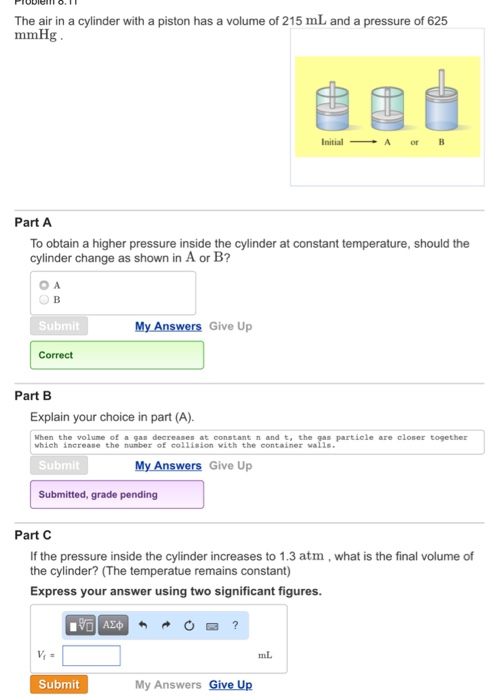The air in a cylinder with a piston has a volume of 215 mL and a pressure of 625 mmHg. To obtain a higher pressure inside the cylinder at constant temperature, should the cylinder change as shown in A or B? Explain your choice in part (A). If the pressure inside the cylinder increases to 1.3 atm, what is the final volume of the cylinder? (The temperatue remains constant) Express your answer using two significant figures.

• ### Problem #4: Air is compressed in a piston cylinder from 1 bar, 17 °C such that...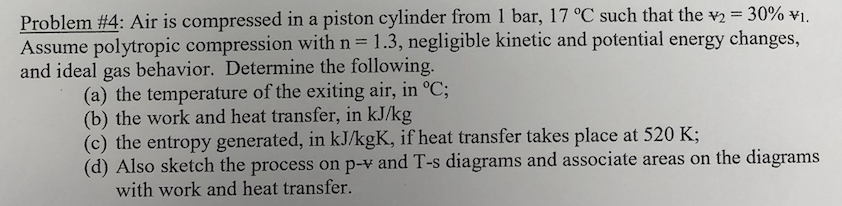Problem #4: Air is compressed in a piston cylinder from 1 bar, 17 °C such that the "2-30% v1 Assume polytropic compression with n - 1.3, negligible kinetic and potential energy changes, and ideal gas behavior. Determine the following. (a) the temperature of the exiting air, in °C; (b) the work and heat transfer, in kJ/kg (c) the entropy generated, in kJ/kgK, if heat transfer takes place at 520 K; (d) Also sketch the process on p-v and T-s diagrams...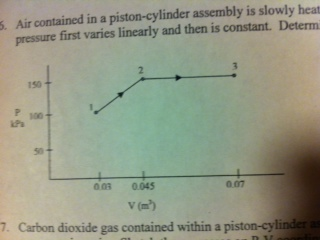Air contained within a piston-cylinder assembly is slowly heated. As shown below, during this process the pressure first varies linearly with volume and then remains constant. Determine the total work, in kJ. 5. Air contained in a piston-cylinder assembly is slowly heat pressure first varies linearly and then is constant. Determ 150 100 LPa 50 003 0.045 007 V(m) 7. Carbon dioxide gas contained within a piston-cy